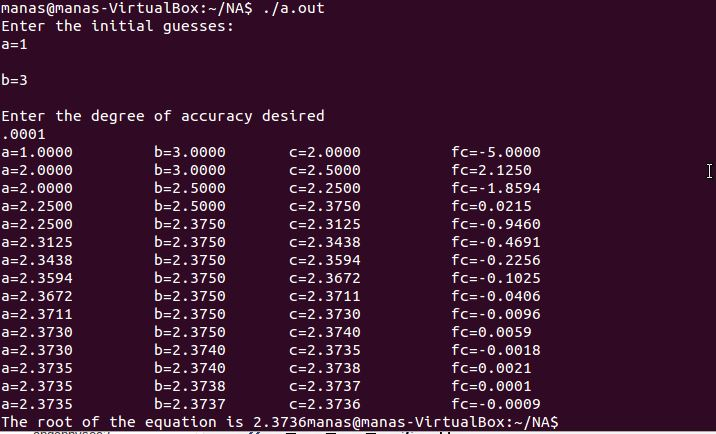# C++ Program for Bisection Method to find the roots of an Equation

```//bisection method
#include<iostream>
#include<cmath>
#include<iomanip>
using namespace std;
double f(double x);    //declare the function for the given equation
double f(double x)    //define the function here, ie give the equation
{
double a=pow(x,3)-x-11.0;    //write the equation whose roots are to be determined
return a;
}
int main()
{
cout.precision(4);        //set the precision
cout.setf(ios::fixed);
double a,b,c,e,fa,fb,fc;    //declare some needed variables
a:cout<<"Enter the initial guesses:\na=";    //Enter the value of a(set a label('a:') for later use with goto)
cin>>a;
cout<<"\nb=";            //Enter the value of b
cin>>b;
cout<<"\nEnter the degree of accuracy desired"<<endl;    //Enter the accuracy
cin>>e;                //e stands for  accuracy
if (f(a)*f(b)>0)        //Check if a root exists between a and b
{                //If f(a)*f(b)>0 then the root does not exist between a and b
cout<<"Please enter a different intial guess"<<endl;
goto a;            //go back to 'a' ie 17 and ask for different values of a and b
}
else                //else a root exists between a and b
{
while (fabs(a-b)>=e)        /*if the mod of a-b is greater than the accuracy desired keep                         bisecting the interval*/
{
c=(a+b)/2.0;        //bisect the interval and find the value of c
fa=f(a);
fb=f(b);
fc=f(c);
cout<<"a="<<a<<"     "<<"b="<<b<<"     "<<"c="<<c<<"      fc="<<fc<<endl;/*print the                             values of a,b,c and fc  after each iteration*/
if (fc==0)        //if f(c)=0, that means we have found the root of the equation
{
cout<<"The root of the equation is "<<c;    /*print the root of the equation                                         and break out of the loop*/
break;
}

if (fa*fc>0)    //if f(a)xf(c)>0, that means no root exist between a and c
{
a=c;    /*hence make a=c, ie make c the starting point of the interval and b the                     end point*/
}
else if (fa*fc<0)
{
b=c;    /*this means that a root exist between a and c therfore make c the end                     point of the interval*/
}

}
}            //The loop ends when the difference between a and b becomes less than the desired accuracy ie now the value stored in 'c' can be called the approximate root of the equation
cout<<"The root of the equation is "<<c;    //print the root
return 0;
}
//output attached as jpg

```Explanation of the above code:[wpedon id="7041" align="center"]

## 9 thoughts on “C++ Program for Bisection Method to find the roots of an Equation”

1. Your loop Has to be do_while.

1. He will write the code the way he wants. Who are you sucker?

2. tnx i like the code but i want to know what did you mean when you say degree of accuracy desired

3. do you have the flow chart in detail for bisection and newton’s raphson method? Please

4. error should be–

Cnew-Cold,,,,, isn’t it?

1. how do i do this

5. sir,what does you mean by degree of accuracy?
Are you talking about iteration or something else?

1. It’s also known as error tolerance.
It implies, that the roots determined at two successive iterations don’t differ more than the degree of accuracy.
This means that the calculations have converged to the tolerance desired. So, for example if you set a tolerance of 0.0001, then the program stops iterating when the root at the current iteration doesn’t differ from the root at the previous iteration by more than 0.0001.
So, this means that the root has converged upto 3 decimal places. So, the numerical root would match the numerical root till 3 decimal places.
A much tighter convergence criteria, implies a very small value of error tolerance, ex: 0.0000001. But this may take a lot of iterations.
Here, is another much rigorous definition: https://en.wikipedia.org/wiki/Order_of_accuracy